# 馈电电路1. 直流电源 $V_{CC}$ 的等效电路应该减小电阻损耗，其等效电路如图(a)
2. 高频基波分量 $I_{cm1}$ 应该通过负载回路输出功率，其等效电路如图(b)
3. 高频谐波分量应该不消耗功率，且不输出，其等效电路如图(c)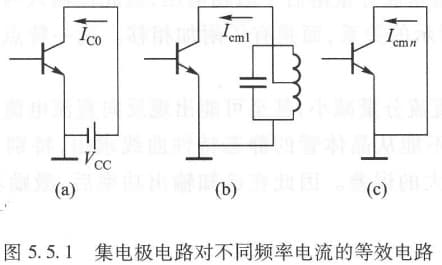## 集电极 串馈/并馈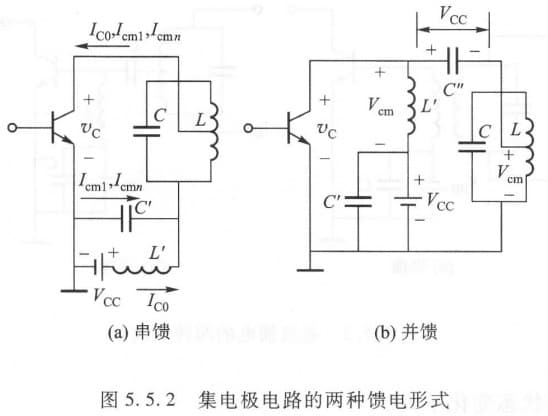## 基极 串馈/并馈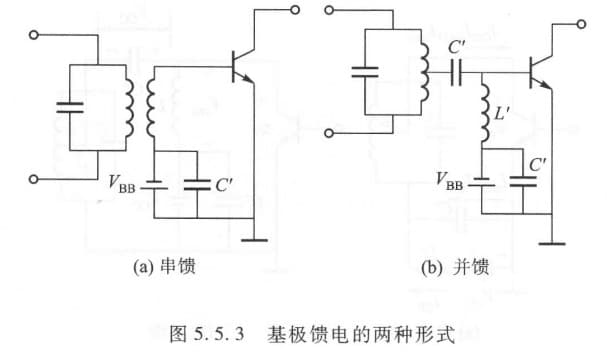$V_{BB}$ 用电池供给并不方便，常采用下面三种方法来获得偏置电压 $V_{BB}$：

1. 利用基极电流的直流分量在 $R_0$ 上产生偏置电压 $V_{BB}$
2. 利用基极电流的直流分量在 $r_{bb’}$ 上产生 $V_{BB}$
3. 利用发射极电流的直流分量在 $R_e$ 上产生 $V_{BB}$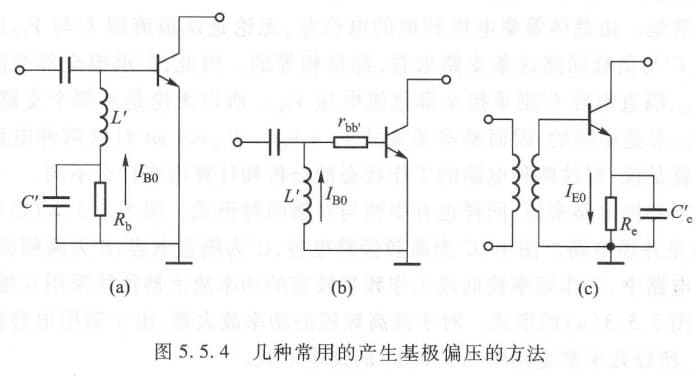# 输出、输入与极间耦合回路

## 输出匹配网络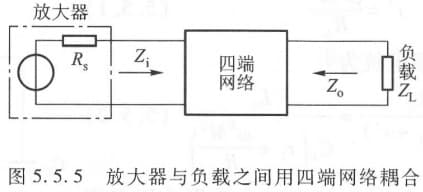1. 负载阻抗与放大器的最佳阻抗相匹配，保证功率最大
2. 抑制工作频率以外的频率，即滤波
3. 有效地传输功率到负载，并且使电子器件彼此隔离，互不影响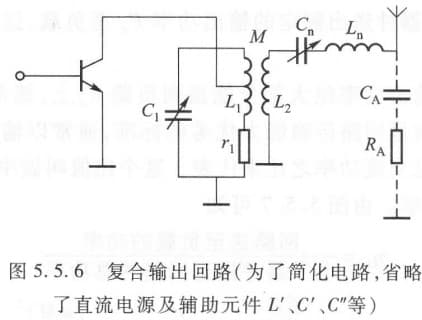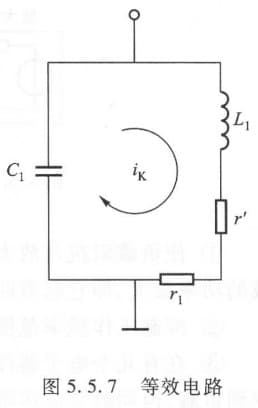$r'=\frac{\omega^2M^2}{R_A}\\ 总电阻 R_p'=\frac{L_1}{C_1(r_1+r')}=\frac{L_1}{C_1\left( r_1+\frac{\omega^2M^2}{R_A} \right)}$

$\eta_k = \frac{回路送到负载的功率}{回路获得的总功率}=\frac{I_k^2 r'}{I_k^2(r_1+r')}=\frac{r'}{r_1+r'}\\ =1-\frac{r_1}{r_1+r'}=1-\frac{R_p'}{R_p}=1-\frac{Q_L}{Q_0}$